Next: Conic Sections Up: Planetary Motion Previous: Conservation Laws

# Polar Coordinates

We can determine the instantaneous position of our planet in the-plane in terms of standard Cartesian coordinates, (,), or polar coordinates, (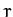,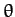), as illustrated in Figure 13. Here,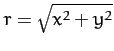and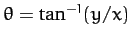. It is helpful to define two unit vectors,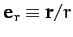and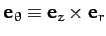, at the instantaneous position of the planet. The first always points radially away from the origin, whereas the second is normal to the first, in the direction of increasing. As is easily demonstrated, the Cartesian components of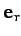and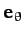are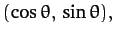(218)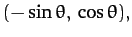(219)

respectively.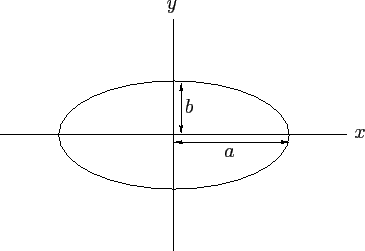We can write the position vector of our planet as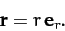(220)

Thus, the planet's velocity becomes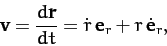(221)

where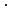is shorthand for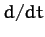. Note thathas a non-zero time-derivative (unlike a Cartesian unit vector) because its direction changes as the planet moves around. As is easily demonstrated, from differentiating Equation (218) with respect to time,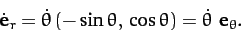(222)

Thus,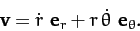(223)

Now, the planet's acceleration is written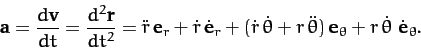(224)

Again,has a non-zero time-derivative because its direction changes as the planet moves around. Differentiation of Equation (219) with respect to time yields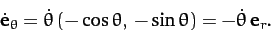(225)

Hence,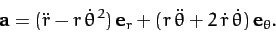(226)

It follows that the equation of motion of our planet, (212), can be written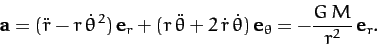(227)

Sinceandare mutually orthogonal, we can separately equate the coefficients of both, in the above equation, to give a radial equation of motion,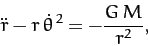(228)

and a tangential equation of motion,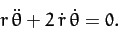(229)Next: Conic Sections Up: Planetary Motion Previous: Conservation Laws
Richard Fitzpatrick 2011-03-31